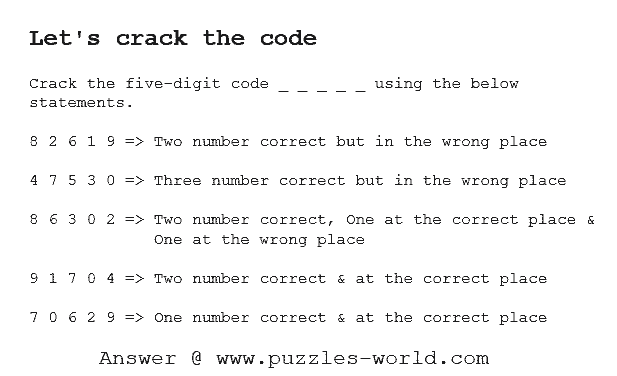# Crack the five digit code 8 2 6 1 9## Let's crack the codeCrack the five digit code _ _ _ _ _ using the below statements.

8 2 6 1 9 => Two number correct but in the wrong place

4 7 5 3 0 => Three number correct but in the wrong place

8 6 3 0 2 => Two number correct, One at the correct place & One at the wrong place

9 1 7 0 4 => Two number correct & at the correct place

7 0 6 2 9 => One number correct & at the correct place

Share with your friends and family and see who is the smartest of them all !!

Explanation :
Let the 5 given statements be A,B,C,D and E
From D and E, we get that 9,7,0 are not part of code,
Hence from D, we get that 1 and 4 are the part of code and in correct position.
also from E, we get that either 6 or 2 is the part of code.

Now from C and above info we get that,
6 and 2 (either one) is part of code and in wrong position for C,
so in C, either 8 or 3 is part of code and in correct position.

Now from A and above info we get that,
8 cannot be part of code since it is in the same position in A and C which would contradict the A statement where it should have been in the wrong place.
So from C we get that 3 is part of code and in correct position.
and hence 6 cannot be part of code since it should be in correct position for E but it would contradict with C where 3 is the position 3 in the code.
So now we have 4 numbers of the code and their position is _ 1 3 2 4.

Now from B, we get that 5,4,3 are in the code since 7 and 0 have been ruled out. So 5 is the final number required to complete the code.

Hence the code is 5 1 3 2 4.